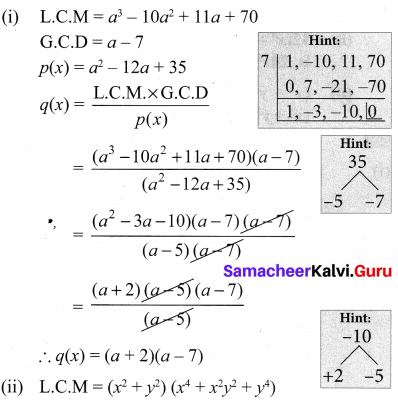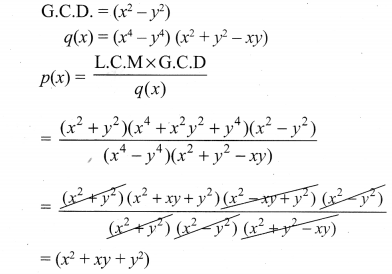# Samacheer Kalvi 10th Maths Solutions Chapter 3 Algebra Ex 3.3

## Tamilnadu Samacheer Kalvi 10th Maths Solutions Chapter 3 Algebra Ex 3.3

10th Maths Exercise 3.3 Samacheer Kalvi Question 1.
Find the LCM and GCD for the following and verify that f(x) × g(x) = LCM × GCD.
(i) 21x2y, 35xy2
(ii) (x3 – 1)(x + 1), x3 + 1
(ii) (x3 – 1) (x + 1), (x3 – 1)
(iii) (x2y + xy2), (x2 + xy)
Solution:
(i) f(x) = 21x2y = 3 × 7x2y
g(x) = 35xy2 = 7 × 5xy2
G.C.D. = 7xy
L.C.M. = 7 × 3 × 5 × x2y2 = 105x2 × y2
L.C.M × G.C.D = f(x) × g(x)
105x2y2 × 7xy = 21x2y × 35xy2
735x3y3 = 735x3y3
Hence verified.

(ii) (x3 – 1)(x + 1) = (x – 1)(x2 + x + 1)(x + 1)
x3 + 1 = (x + 1) (x2 – x + 1)
G.C.D = (x+ 1)
L.C.M = (x – 1)(x + 1)(x2 + x + 1)(x2 – x + 1)
∴ L.C.M. × G.C.D = f(x) × g(x)
(x – 1)(x + 1)(x2 + x + 1) (x2 – x + 1) = (x – 1)
(x2 + x + 1) × (x + 1) (x2 – x + 1)
(x3 – 1)(x + 1)(x3 + 1) = (x3 – 1)(x + 1)(x3 + 1)
∴ Hence verified.

(iii) f(x) = x2y + xy2 = xy(x + y)
g(x) = x2 + xy = x(x + y)
L.C.M. = x y (x + y)
G.C.D. = x (x + y)
To verify:
L.C.M. × G.C.D. = xy(x + y) × (x + y)
= x2y (x + y)2 ……….. (1)
f(x) × g (x) = (x2y + xy2)(x2 + xy)
= x2y (x + y)2 …………… (2)
∴ L.C.M. × G.C.D = f(x) × g{x).
Hence verified.

Ex 3.3 Class 10 Samacheer Question 2.
Find the LCM of each pair of the following polynomials
(i) a2 + 4a – 12, a2 – 5a + 6 whose GCD is a – 2
(ii) x4 – 27a3x, (x – 3a)2 whose GCD is (x – 3a)
Solution:
(i) f(x) = a2 + 4a – 12 = (a + 6)(a – 2)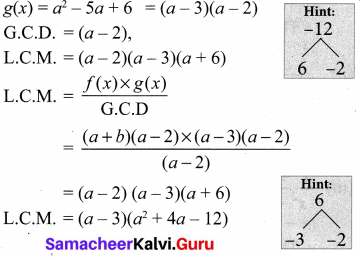(ii) f(x) = x4 – 27a3x = x(x3 – (3a)3)
g(x) = (x – 3a)2
G.C.D = (x – 3a)
L.C.M. × G.C.D = f(x) × g(x)
L. C.M = $$\frac{x\left(x^{3}-(3 a)^{3}\right) \times(x-3 a)^{2}}{(x-3 a)}$$
L.C.M = x(x3 – (3a)3) . (x – 3a)
= x(x – 3a)2 (x2 + 3ax + 9a2)

10th Maths Exercise 3.3 Question 3.
Find the GCD of each pair of the following polynomials
(i) 12(x4 – x3), 8(x4 – 3x3 + 2x2) whose LCM is 24x3 (x – 1)(x – 2)
(ii) (x3 + y3), (x4 + x2y2 + y4) whose LCM is (x3 + y3) (x2 + xy + y2)
Solution:
(i) f(x)= 12(x4 – x3)
g(x) = 8(x4 – 3x3 + 2x2)
L.C.M = 24x3 (x – 1)(x – 2)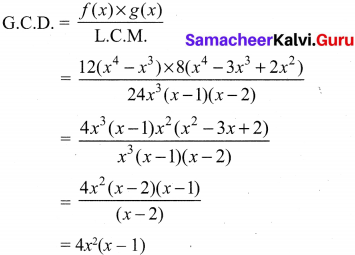(ii) (x3 + y3), (x4 + x2y2 + y4)
L.C.M. = (x3 + y3)(x2 + xy + y2)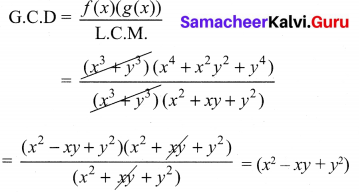10th Maths 3.3 Exercise Samacheer Kalvi Question 4.
Given the LCM and GCD of the two polynomials p(x) and q(x) find the unknown polynomial in the following table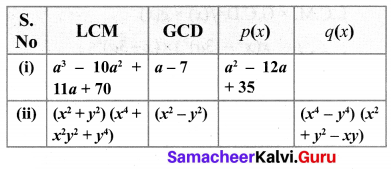Solution: